Refer to our Texas Go Math Grade 2 Answer Key Pdf to score good marks in the exams. Test yourself by practicing the problems from Texas Go Math Grade 2 Module 16 Assessment Answer Key.

Vocabulary

Use words from the box to complete the sentence.

Question 1.
A _________________ is the same length as 12 inches. (p. 558)
A foot is the same length as 12 inches.

An _________________ is shorter than a foot. (p.546)
An inch is shorter than a foot.

Concepts and Skills

Question 3.
Use color tiles. Measure the length of the object in inches. TEKS 2.9.A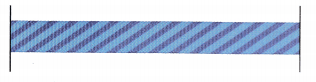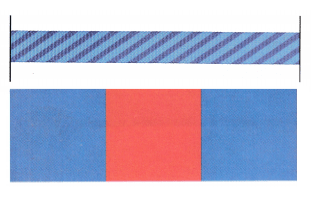3 inches

Explanation:
The length of the ribbon is 3 color tiles
Each color tile is 1 inch, and the ribbon is 3 inches long.

Use a ruler. Measure to the nearest inch. Then measure to the nearest foot. TEKS 2.9.D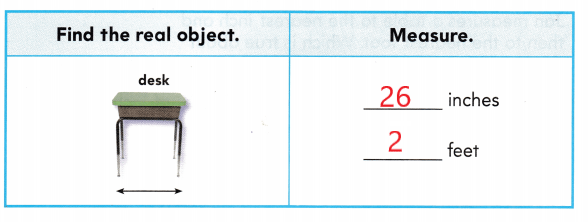Explanation:
length in inches 26 inches
length in feet 2 feet
The length of the desk is about 26 inches and about 2 feet.

Text Test Prep

Fill in the bubble for the correct answer choice.

Question 5.
Bert estimates the length of a real bulletin board. Which is the best estimate? TEKS 2.9.E(A) 3 inches
(B) 30 inches
(C) 12 inches
24 inches
2 feet

Explanation:
The length of the bulletin board is about 24 inches and about 2 feet.

Jennie wants to measure the length of her pen in inches. Which is the best tool to use? TEKS 2.9.D.
(A) yardstick
(B) inch ruler
(C) piece of string
B. Inch ruler

Explanation:
An inch ruler tells the measurement of the objects in inches.

Question 7.
Use an inch ruler. What is the length of the crayon to the nearest inch? TEKS 2.9.D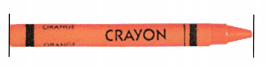(A) 4 inches
(B) 1 inch
(C) 3 inches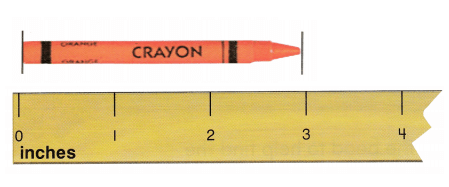Explanation:
I lines up the crayon along the inch ruler
I marked the end of the crayon that is 3 inches
Therefore, the crayon is about 3 inches long.